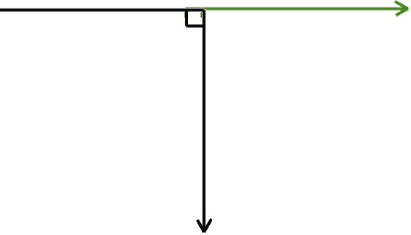# Magnetic field of infinite wire

## Homework Statement

An infinite wire carries current I.I hope the picture works! The vertical arrow shows the direction of current in the wire. The green arrow has length a and ends at point P. What is the magnitude of the magnetic field at point P?

## Homework Equations

dB = (μ/4π) (I dl×r )/r3
Where the r on the numerator is a position vector.

## The Attempt at a Solution

The horizontal part of the wire is irrelevant, as l is in the same direction as r at that point.

r is the distance of P from the vertical wire, I think. I called the direction of the green arrow the positive x direction, the direction of current and of dl is the y direction. I have to find the magnitude of the field, so I have to integrate the expression for dB. dl can be replaced with dy as they are in the same direction.

I, μ and 4π are constants and can be taken out of an integral. The magnitude of the cross product on the numerator is given by r dy sinφ, where r now cancels with the r in the denominator.
I have to do (μI / 4π) ∫ (dy sinφ) / r2
So the distance r to the wire will be given by Pythagoras' theorem, so r = (x2+y2)1/2.
My major problem is, I don't really know which angle φ is. It's one of two! Is sinφ given by x/(x2+y2) or should y be on the numerator instead?

Anyway, I carried on assuming x is on the numerator. Then the integral becomes:
∫ x dy / (x2+y2)3/2

So do a substitution of y=xtanφ. And then how I carry on really depends on what angle φ is!
Thanks for any help :)

Last edited: